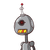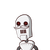# What should be added to −5by 4– to get -1?options are(i) −1by4(ii) 1 December 17, 2021 by Camila

What should be added to −5by 4

– to get -1?
options are

(i) −1

by4

(ii) 1

by4

(iii) 1
(iv) −3

by 4​

### 2 thoughts on “What should be added to −5by 4<br /><br />– to get -1?<br />options are<br /><br />(i) −1<br /><br />by4<br /><br />(ii) 1<br /><b”

1.3rd option 1

Step-by-step explanation:

‐5/4+1=-5/4+1/4

=-5/4+4/4=-1/4

2.$$\frac{ – 5}{4} + x = – 1 \\ = > x = – 1 + \frac{5}{4} \\ = > x = \frac{ – 4 + 5}{4} \\ = > x = \frac{ – 1}{4}$$